#### 期刊菜单

A Method for Locating Disconnected Faults in Distribution Networks Based on Negative Sequence Voltage
DOI: 10.12677/SG.2022.121001, PDF, HTML, XML, 下载: 175  浏览: 307

Abstract: With the continuous expansion of the scale of the distribution network, the insulation rate of overhead lines has increased, and the incidence of disconnection faults has also increased. Due to the disconnection fault, the three-phase voltage and current are seriously unbalanced, which endangers electrical equipment and personal safety, and affects the reliability of the power supply of the power system. Therefore, it is necessary to conduct research on the method of locating disconnection faults in the distribution network, and to investigate and isolate them as soon as possible after the disconnection fault occurs, so as to reduce the harm. This paper considers the influence of distributed generations, and analyzes the negative sequence voltage characteristics of distributed generations under the disconnection fault of the distribution network. On this basis, according to the difference in sequence voltage of distributed generations under different fault locations, a method for locating disconnection faults in distribution network based on negative sequence voltage is proposed. The simulation results show that the proposed method can accurately locate the disconnection fault section and has high reliability.

1. 引言

2. 配电网断线故障特征

${i}_{D+,f}=\frac{{S}_{D}}{{u}_{D+,f}}$ (1)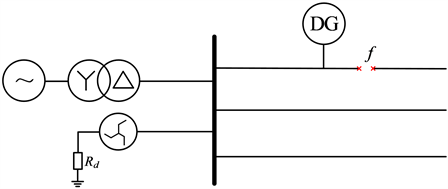Figure 1. Schematic diagram of disconnection fault of distribution network

$\left\{\begin{array}{l}{{\stackrel{˙}{I}}^{\prime }}_{\text{A}}=0\\ \Delta {{\stackrel{˙}{U}}^{\prime }}_{\text{B}}=\Delta {{\stackrel{˙}{U}}^{\prime }}_{\text{C}}\end{array}$ (2)

$\left\{\begin{array}{l}{{\stackrel{˙}{I}}^{\prime }}_{\text{A}1}+{{\stackrel{˙}{I}}^{\prime }}_{\text{A}2}+{{\stackrel{˙}{I}}^{\prime }}_{\text{A}0}=0\\ \Delta {{\stackrel{˙}{U}}^{\prime }}_{\text{A}1}=\Delta {{\stackrel{˙}{U}}^{\prime }}_{\text{A}2}=\Delta {{\stackrel{˙}{U}}^{\prime }}_{\text{A}0}\end{array}$ (3)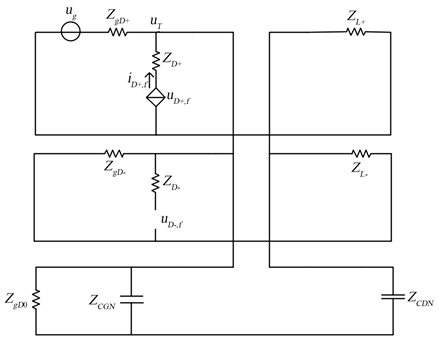Figure 2. Equivalent composite sequence network of disconnection fault in distribution network with distributed generation

$\left\{\begin{array}{l}{u}_{g}={u}_{T}+\left(\frac{{u}_{g}-{u}_{T}}{{Z}_{gD+}}+{i}_{D+,f}\right)\left[{Z}_{L+}+\left({Z}_{L-}+{Z}_{gD-}\right)\parallel \left({Z}_{gD0}\parallel {Z}_{CGN}+{Z}_{CDN}\right)\right]\\ {u}_{D+,f}={i}_{D+,f}{Z}_{D+}+\left(\frac{{u}_{g}-{u}_{T}}{{Z}_{gD+}}+{i}_{D+,f}\right)\left[{Z}_{L+}+\left({Z}_{L-}+{Z}_{gD-}\right)\parallel \left({Z}_{gD0}\parallel {Z}_{CGN}+{Z}_{CDN}\right)\right]\\ {u}_{T}={u}_{D+,f}-{i}_{D+,f}{Z}_{D+}\\ {u}_{D-,f}=\frac{{u}_{T}\left({Z}_{L-}+{Z}_{gD-}\right)\parallel \left({Z}_{gD0}\parallel {Z}_{CGN}+{Z}_{CDN}\right)}{\left({Z}_{L-}+{Z}_{gD-}\right)\parallel \left({Z}_{gD0}\parallel {Z}_{CGN}+{Z}_{CDN}\right)+{Z}_{L+}}\cdot \frac{{Z}_{gD-}}{{Z}_{gD-}+{Z}_{L-}}\end{array}$ (4)

${u}_{D-,f}=\frac{{u}_{T}}{\left({Z}_{gD-}+{Z}_{L-}\right)\parallel \left({Z}_{gD0}\parallel {Z}_{CGN}+{Z}_{CDN}\right)+{Z}_{L+}}\cdot \frac{\left({Z}_{gD0}\parallel {Z}_{CGN}+{Z}_{CDN}\right){Z}_{gD-}}{\left({Z}_{gD0}\parallel {Z}_{CGN}+{Z}_{CDN}\right)+{Z}_{gD-}+{Z}_{L-}}$ (5)

${u}_{T}=\frac{2\left({Z}_{gD+}+{Z}_{D+}\right){S}_{D}}{-{u}_{g}+\sqrt{{u}_{g}^{2}+4\left({Z}_{gD+}+{Z}_{D+}\right){S}_{D}}}-\frac{-{u}_{g}+\sqrt{{u}_{g}^{2}+4\left({Z}_{gD+}+{Z}_{D+}\right){S}_{D}}}{2\left({Z}_{gD+}+{Z}_{D+}\right)}{Z}_{D+}$ (6)

3. 基于负序电压的配电网断线故障定位方法

3.1. 定位原理

${u}_{i,st}=\frac{{H}_{i}}{{Z}_{gD-}+{Z}_{L-}+{Z}_{L+}}\cdot {Z}_{gD-}$ (7)

${H}_{i}=\frac{2\left({Z}_{gD}+{Z}_{i,m}\right){S}_{i,m}^{\mathrm{min}}}{-{u}_{g}+\sqrt{{u}_{g}^{2}+4\left({Z}_{gD}+{Z}_{i,m}\right){S}_{i,m}^{\mathrm{min}}}}-\frac{-{u}_{g}+\sqrt{{u}_{g}^{2}+4\left({Z}_{gD}+{Z}_{i,m}\right){S}_{i,m}^{\mathrm{min}}}}{2\left({Z}_{gD}+{Z}_{i,m}\right)}{Z}_{i,m}$ (8)

${U}_{ij,op}^{t}=\frac{{M}_{ij}}{\left({Z}_{gD-}+{Z}_{L-}\right)\parallel \left({Z}_{gD0}\parallel {Z}_{CGN}+{Z}_{CDN}\right)+{Z}_{L+}}\cdot \frac{\left({Z}_{gD0}\parallel {Z}_{CGN}+{Z}_{CDN}\right){Z}_{gD-}}{\left({Z}_{gD0}\parallel {Z}_{CGN}+{Z}_{CDN}\right)+{Z}_{gD-}+{Z}_{L-}}$ (9)

${M}_{ij}=\frac{2\left({Z}_{gD}+{Z}_{i,j}\right){S}_{ij}^{t}}{-{u}_{g}+\sqrt{{u}_{g}^{2}+4\left({Z}_{gD}+{Z}_{i,j}\right){S}_{ij}^{t}}}-\frac{-{u}_{g}+\sqrt{{u}_{g}^{2}+4\left({Z}_{gD}+{Z}_{i,j}\right){S}_{ij}^{t}}}{2\left({Z}_{gD}+{Z}_{i,j}\right)}{Z}_{i,j}$ (10)

3.2. 定位方法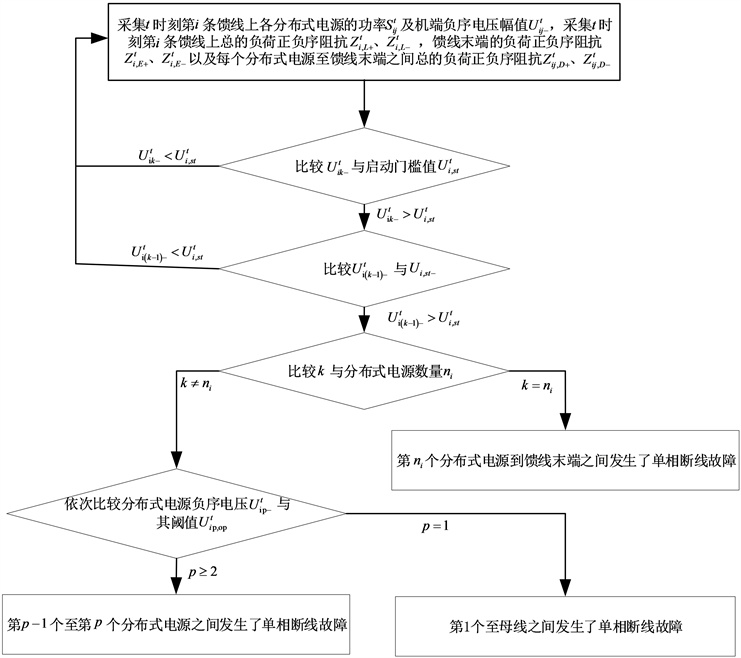Figure 3. A method for locating disconnected faults in distribution networks based on negative sequence voltage

4. 仿真与分析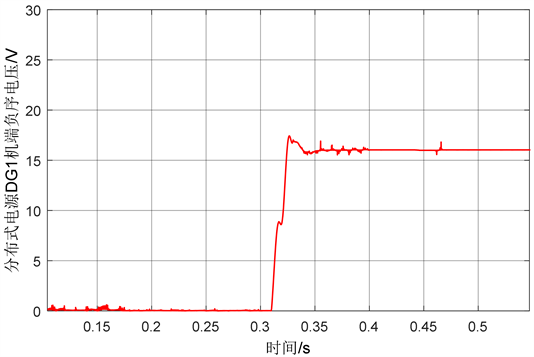Figure 4. DG1 terminal negative sequence voltage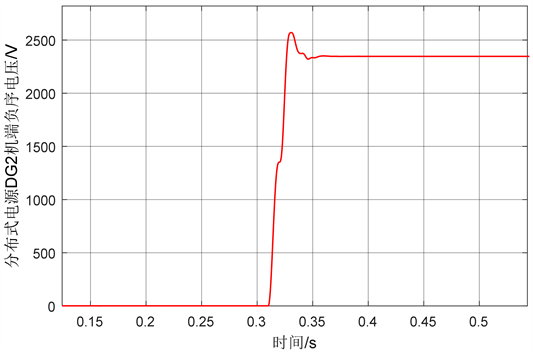Figure 5. DG2 terminal negative sequence voltage

5. 结论

  贾巍, 雷才嘉, 葛磊蛟, 高慧. 城市配电网的国内外发展综述及技术展望[J]. 电力电容器与无功补偿, 2020, 41(1): 158-168+175. https://doi.org/10.14044/j.1674-1757.pcrpc.2020.01.026  肖勇, 陆文升, 李云涛, 钟玉廷, 亓彦珣, 彭家颖. 城市配电网发展形态指标体系及其评估方法研究[J]. 电力系统保护与控制, 2021, 49(1): 62-71. https://doi.org/10.19783/j.cnki.pspc.200803  杜洋, 杨心刚, 郭灵瑜, 王玉洁, 周静涵, 谭忠富. 双碳背景下配电网绿色发展与安全高效耦合评价[J]. 科学技术与工程, 2021, 21(30): 12973-12981.  韩肖清, 李廷钧, 张东霞, 周鑫. 双碳目标下的新型电力系统规划新问题及关键技术[J]. 高电压技术, 2021, 47(9): 3036-3046. https://doi.org/10.13336/j.1003-6520.hve.20210809  王鲍雅琼, 陈皓. 含分布式电源的配电网保护改进方案综述[J]. 电力系统保护与控制, 2017, 45(12): 146-154.  伦惠勤, 王佳, 胡扬, 赵崇, 胡剑锋. 智能配电网研究现状及发展展望[J]. 电测与仪表, 2015, 52(8): 10-15.  刘小春, 王巍璋, 姚文昊, 周成, 王淳, 江涛. 中压配电网故障处理模式配置研究综述[J]. 电测与仪表, 2021, 58(10): 49-58. https://doi.org/10.19753/j.issn1001-1390.2021.10.008  全蕾, 詹红霞, 张勇, 肖竣文, 彭平. 考虑多DG接入的配电网自适应电流主保护方案[J]. 智慧电力, 2021, 49(8): 63-69.  康党兴, 李萌. 10 kV配电网电压异常现象及对策[J]. 农村电工, 2020, 28(12): 38-39. https://doi.org/10.16642/j.cnki.ncdg.2020.12.043  孟祥忠, 陈文聪, 李金宝. 中压配电网单相断线故障检测及定位的研究[J]. 电子测量技术, 2020, 43(6): 32-37. https://doi.org/10.19651/j.cnki.emt.1903531  薛永端, 陈梦琦, 曹丽丽, 冯光, 徐丙垠. 不接地系统单相断线故障电压分析[J]. 中国电机工程学报, 2021, 41(4): 1322-1333+1539. https://doi.org/10.13334/j.0258-8013.pcsee.191665  王成楷, 洪志章. 基于线电压判据的配电网单相断线故障定位方法[J]. 电气技术, 2017(5): 51-57.  禹红, 郑泷康, 曾荷清, 李付亮. 小水电发电系统的非接地电网单相断线故障分析及诊断方法[J]. 湖南电力, 2020, 40(2): 1-7.  刘健, 张志华, 王毅钊. 基于电压信息的配电网断线故障定位[J]. 电力系统自动化, 2020, 44(21): 123-131.  盛方正, 陈子聪, 陈晓炯. 中性点不接地系统单相断线故障分析及对策研究[J]. 供用电, 2011, 28(3): 43-45.  盛方正, 陈福良, 范秉翰, 王文成. 小电阻接地系统单相断线故障分析及对策研究[J]. 浙江电力, 2011, 30(7): 6-9+25. https://doi.org/10.19585/j.zjdl.2011.07.002  朱玲玲, 李长凯, 张华中, 张忠. 配电网单相断线故障负序电流分析及选线[J]. 电力系统保护与控制, 2009, 37(9): 35-38+43.  康奇豹, 丛伟, 盛亚如, 王玥婷. 配电线路单相断线故障保护方法[J]. 电力系统保护与控制, 2019, 47(8): 127-136.  常仲学, 宋国兵, 张维. 配电网单相断线故障的负序电压电流特征分析及区段定位[J]. 电网技术, 2020, 44(8): 3065-3074. https://doi.org/10.13335/j.1000-3673.pst.2019.1830  王玥婷, 梁中会, 牟欣玮, 王邦惠, 鲍忠伟, 丛志鹏, 张颖, 史振字. 考虑分布式能源的配电网断线定位方法[J]. 电力系统保护与控制, 2018, 46(21): 131-137.  杜雪, 欧阳金鑫, 龙晓轩, 等. 基于分布式电源电流变化率的主动配电网单相断线保护方法[J]. 电力系统保护与控制, 2020, 48(22): 41-48.